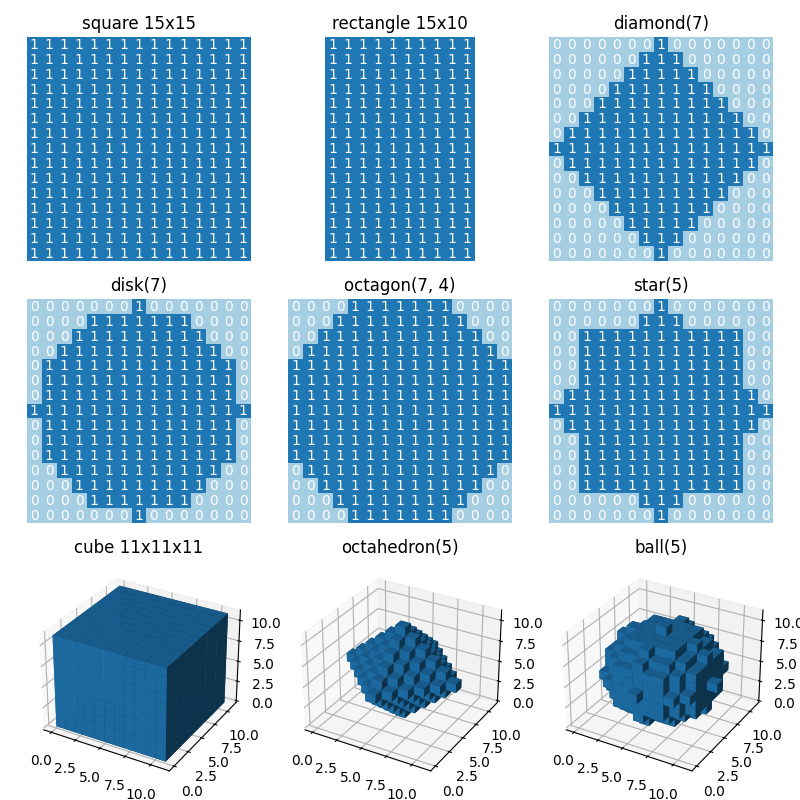# Generate structuring elements¶

This example shows how to use functions in skimage.morphology to generate structuring elements. The title of each plot indicates the call of the function.import numpy as np
import matplotlib.pyplot as plt
from mpl_toolkits.mplot3d import Axes3D

from skimage.morphology import (square, rectangle, diamond, disk, cube,
octahedron, ball, octagon, star)

# Generate 2D and 3D structuring elements.
struc_2d = {
"square(15)": square(15),
"rectangle(15, 10)": rectangle(15, 10),
"diamond(7)": diamond(7),
"disk(7)": disk(7),
"octagon(7, 4)": octagon(7, 4),
"star(5)": star(5)
}

struc_3d = {
"cube(11)": cube(11),
"octahedron(5)": octahedron(5),
"ball(5)": ball(5)
}

# Visualize the elements.
fig = plt.figure(figsize=(8, 8))

idx = 1
for title, struc in struc_2d.items():
ax.imshow(struc, cmap="Paired", vmin=0, vmax=12)
for i in range(struc.shape):
for j in range(struc.shape):
ax.text(j, i, struc[i, j], ha="center", va="center", color="w")
ax.set_axis_off()
ax.set_title(title)
idx += 1

for title, struc in struc_3d.items():
ax = fig.add_subplot(3, 3, idx, projection=Axes3D.name)
ax.voxels(struc)
ax.set_title(title)
idx += 1

fig.tight_layout()
plt.show()


Total running time of the script: ( 0 minutes 1.718 seconds)

Gallery generated by Sphinx-Gallery